# forecast

Forecast vector autoregression (VAR) model responses

## Syntax

``Y = forecast(Mdl,numperiods,Y0)``
``Y = forecast(Mdl,numperiods,Y0,Name,Value)``
``````[Y,YMSE] = forecast(___)``````

## Description

example

````Y = forecast(Mdl,numperiods,Y0)` returns a path of minimum mean squared error (MMSE) forecasts (`Y`) over the length `numperiods` forecast horizon using the fully specified VAR(p) model `Mdl`. The forecasted responses represent the continuation of the presample data `Y0`.```

example

````Y = forecast(Mdl,numperiods,Y0,Name,Value)` uses additional options specified by one or more name-value arguments. For example, you can specify future exogenous predictor data or include future responses for conditional forecasting. ```

example

``````[Y,YMSE] = forecast(___)``` returns the corresponding mean squared error (MSE) of each forecasted response using any of the input arguments in the previous syntaxes.```

## Examples

collapse all

Fit a VAR(4) model to the consumer price index (CPI) and unemployment rate data. Then, forecast unconditional MMSE responses from the estimated model.

Load the `Data_USEconModel` data set.

`load Data_USEconModel`

Plot the two series on separate plots.

```figure; plot(DataTable.Time,DataTable.CPIAUCSL); title('Consumer Price Index'); ylabel('Index'); xlabel('Date');``````figure; plot(DataTable.Time,DataTable.UNRATE); title('Unemployment Rate'); ylabel('Percent'); xlabel('Date');```Stabilize the CPI by converting it to a series of growth rates. Synchronize the two series by removing the first observation from the unemployment rate series.

```rcpi = price2ret(DataTable.CPIAUCSL); unrate = DataTable.UNRATE(2:end); Data = array2timetable([rcpi unrate],'RowTimes',DataTable.Time(2:end),... 'VariableNames',{'rcpi','unrate'});```

Create a default VAR(4) model using the shorthand syntax.

`Mdl = varm(2,4);`

Estimate the model using the entire data set.

`EstMdl = estimate(Mdl,Data.Variables);`

`EstMdl` is a fully specified, estimated `varm` model object.

Forecast responses from the estimated model over a three-year horizon. Specify the entire data set as presample observations.

```numperiods = 12; Y0 = Data.Variables; Y = forecast(EstMdl,numperiods,Y0);```

`Y` is a 12-by-2 matrix of forecasted responses. The first and second columns contain the simulated CPI growth rate and unemployment rate, respectively.

Plot the forecasted responses and the last 50 true responses.

```fh = dateshift(Data.Time(end),'end','quarter',1:12); figure; h1 = plot(Data.Time((end-49):end),Data.rcpi((end-49):end)); hold on; h2 = plot(fh,Y(:,1)); title('CPI Growth Rate'); ylabel('Growth rate'); xlabel('Date'); h = gca; fill([Data.Time(end) fh([end end]) Data.Time(end)],h.YLim([1 1 2 2]),'k',... 'FaceAlpha',0.1,'EdgeColor','none'); legend([h1 h2],'True','Forecast') hold off;```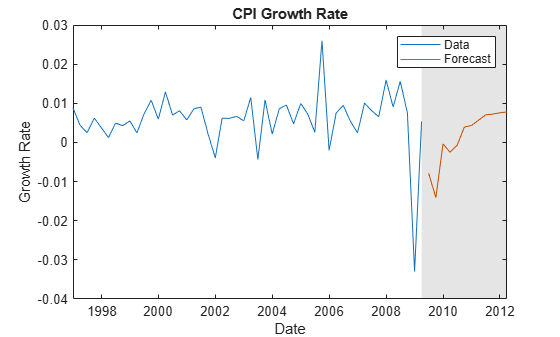```figure; h1 = plot(Data.Time((end-49):end),Data.unrate((end-49):end)); hold on; h2 = plot(fh,Y(:,2)); title('Unemployment Rate'); ylabel('Percent'); xlabel('Date'); h = gca; fill([Data.Time(end) fh([end end]) Data.Time(end)],h.YLim([1 1 2 2]),'k',... 'FaceAlpha',0.1,'EdgeColor','none'); legend([h1 h2],'True','Forecast','Location','northwest') hold off;```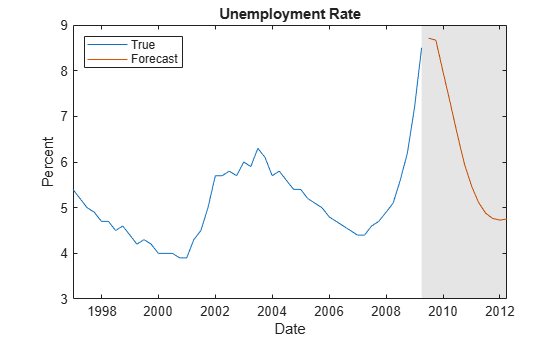Estimate a four-degree vector autoregression model including exogenous predictors (VARX(4)) of the consumer price index (CPI), the unemployment rate, and the gross domestic product (GDP). Include a linear regression component containing the current quarter and the last four quarters of government consumption expenditures and investment (GCE). Forecast a response path from the estimated model.

Load the `Data_USEconModel` data set. Compute the real GDP.

```load Data_USEconModel DataTable.RGDP = DataTable.GDP./DataTable.GDPDEF*100;```

Plot all variables on separate plots.

```figure; subplot(2,2,1) plot(DataTable.Time,DataTable.CPIAUCSL); ylabel('Index'); title('Consumer Price Index'); subplot(2,2,2) plot(DataTable.Time,DataTable.UNRATE); ylabel('Percent'); title('Unemployment Rate'); subplot(2,2,3) plot(DataTable.Time,DataTable.RGDP); ylabel('Output'); title('Real Gross Domestic Product'); subplot(2,2,4) plot(DataTable.Time,DataTable.GCE); ylabel('Billions of \$'); title('Government Expenditures');```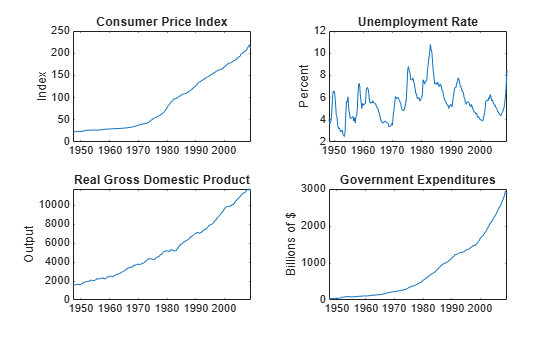Stabilize the CPI, GDP, and GCE by converting each to a series of growth rates. Synchronize the unemployment rate series with the others by removing its first observation.

```inputVariables = {'CPIAUCSL' 'RGDP' 'GCE'}; Data = varfun(@price2ret,DataTable,'InputVariables',inputVariables); Data.Properties.VariableNames = inputVariables; Data.UNRATE = DataTable.UNRATE(2:end);```

Expand the GCE rate series to a matrix that includes its current value and up through four lagged values. Remove the `GCE` variable from `Data`.

```rgcelag4 = lagmatrix(Data.GCE,0:4); Data.GCE = [];```

Create a default VAR(4) model using the shorthand syntax.

```Mdl = varm(3,4); Mdl.SeriesNames = ["rcpi" "unrate" "rgdpg"];```

Estimate the model using all but the last three years of data. Specify the GCE matrix as data for the regression component.

```bfh = Data.Time(end) - years(3); estIdx = Data.Time < bfh; EstMdl = estimate(Mdl,Data{estIdx,:},'X',rgcelag4(estIdx,:));```

Forecast a path of quarterly responses three years into the future.

```Y0 = Data{estIdx,:}; Y = forecast(EstMdl,12,Data{estIdx,:},'X',rgcelag4(~estIdx,:));```

`Y` is a 12-by-3 matrix of simulated responses. The columns correspond to the CPI growth rate, unemployment rate, and real GDP growth rate, respectively.

Plot the forecasted responses and the last 50 true responses.

```figure; for j = 1:Mdl.NumSeries subplot(2,2,j) h1 = plot(Data.Time((end-49):end),Data{(end-49):end,j}); hold on; h2 = plot(Data.Time(~estIdx),Y(:,j)); title(Mdl.SeriesNames{j}); h = gca; fill([bfh h.XLim([2 2]) bfh],h.YLim([1 1 2 2]),'k',... 'FaceAlpha',0.1,'EdgeColor','none'); hold off; end hl = legend([h1 h2],'Data','Forecast'); hl.Position = [0.6 0.25 hl.Position(3:4)];```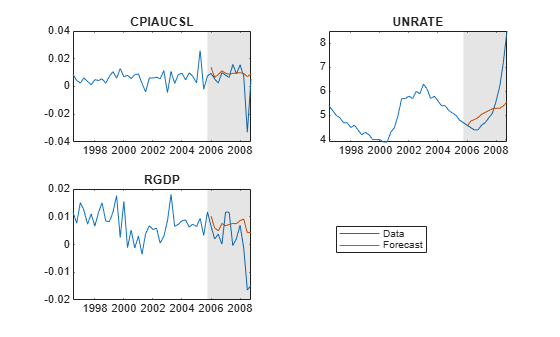Analyze forecast accuracy using forecast intervals over a three-year horizon. This example follows from Forecast Unconditional Response Series from VAR(4) Model.

Load the `Data_USEconModel` data set and preprocess the data.

```load Data_USEconModel rcpi = price2ret(DataTable.CPIAUCSL); unrate = DataTable.UNRATE(2:end); Data = array2timetable([rcpi unrate],'RowTimes',DataTable.Time(2:end),... 'VariableNames',{'rcpi','unrate'});```

Estimate a VAR(4) model of the two response series. Reserve the last three years of data.

```bfh = Data.Time(end) - years(3); estIdx = Data.Time < bfh; Mdl = varm(2,4); EstMdl = estimate(Mdl,Data{estIdx,:});```

Forecast responses from the estimated model over a three-year horizon. Specify the entire data set as presample observations. Return the MSE of the forecasts.

```numperiods = 12; Y0 = Data{estIdx,:}; [Y,YMSE] = forecast(EstMdl,numperiods,Y0);```

`Y` is a 12-by-2 matrix of forecasted responses. `YMSE` is a 12-by-1 cell vector of corresponding MSE matrices.

Extract the main diagonal elements from the matrices in each cell of `YMSE`. Apply the square root of the result to obtain standard errors.

```extractMSE = @(x)diag(x)'; MSE = cellfun(extractMSE,YMSE,'UniformOutput',false); SE = sqrt(cell2mat(MSE));```

Estimate approximate 95% forecast intervals for each response series.

```YFI = zeros(numperiods,Mdl.NumSeries,2); YFI(:,:,1) = Y - 2*SE; YFI(:,:,2) = Y + 2*SE;```

Plot the forecasted responses and the last 50 true responses.

```figure; h1 = plot(Data.Time((end-49):end),Data.rcpi((end-49):end)); hold on; h2 = plot(Data.Time(~estIdx),Y(:,1)); h3 = plot(Data.Time(~estIdx),YFI(:,1,1),'k--'); plot(Data.Time(~estIdx),YFI(:,1,2),'k--'); title('CPI Growth Rate'); ylabel('Growth rate'); xlabel('Date'); h = gca; fill([bfh h.XLim([2 2]) bfh],h.YLim([1 1 2 2]),'k',... 'FaceAlpha',0.1,'EdgeColor','none'); legend([h1 h2 h3],'True','Forecast','95% Forecast interval',... 'Location','northwest') hold off;```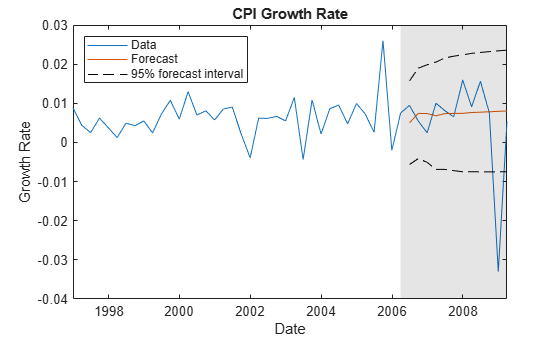```figure; h1 = plot(Data.Time((end-49):end),Data.unrate((end-49):end)); hold on; h2 = plot(Data.Time(~estIdx),Y(:,2)); h3 = plot(Data.Time(~estIdx),YFI(:,2,1),'k--'); plot(Data.Time(~estIdx),YFI(:,2,2),'k--'); title('Unemployment Rate'); ylabel('Percent'); xlabel('Date'); h = gca; fill([bfh h.XLim([2 2]) bfh],h.YLim([1 1 2 2]),'k',... 'FaceAlpha',0.1,'EdgeColor','none'); legend([h1 h2 h3],'True','Forecast','95% Forecast interval',... 'Location','northwest') hold off;```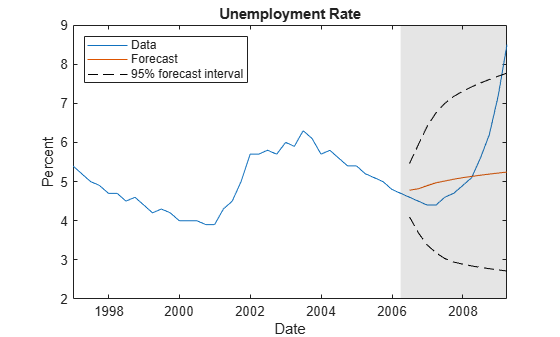## Input Arguments

collapse all

VAR model, specified as a `varm` model object created by `varm` or `estimate`. `Mdl` must be fully specified.

Forecast horizon, or the number of time points in the forecast period, specified as a positive integer.

Data Types: `double`

Presample responses that provide initial values for the forecasts, specified as a `numpreobs`-by-`numseries` numeric matrix or a `numpreobs`-by-`numseries`-by-`numprepaths` numeric array.

`numpreobs` is the number of presample observations. `numseries` is the number of response series (`Mdl.NumSeries`). `numprepaths` is the number of presample response paths.

Rows correspond to presample observations, and the last row contains the latest observation. `Y0` must contain at least `Mdl.P` rows. If you supply more rows than necessary, `forecast` uses only the latest `Mdl.P` observations.

Columns must correspond to the response series names in `Mdl.SeriesNames`.

Pages correspond to separate, independent paths.

• If you do not specify the `YF` name-value pair argument, then `forecast` initializes each forecasted path (page) using the corresponding page of `Y0`. Therefore, the output argument `Y` has `numprepaths` pages.

• If you specify the `YF` name-value pair argument, then `forecast` takes one of these actions.

• If `Y0` is a matrix, then `forecast` initializes each forecast path (page) in `YF` using `Y0`. Therefore, all paths in the output argument `Y` derive from common initial conditions.

• Otherwise, `forecast` applies `Y0(:,:,j)` to initialize forecasting path `j`. `Y0` must have at least `numpaths` pages, and `forecast` uses only the first `numpaths` pages.

Among all pages, observations in a particular row occur at the same time.

Data Types: `double`

### Name-Value Arguments

Specify optional pairs of arguments as `Name1=Value1,...,NameN=ValueN`, where `Name` is the argument name and `Value` is the corresponding value. Name-value arguments must appear after other arguments, but the order of the pairs does not matter.

Before R2021a, use commas to separate each name and value, and enclose `Name` in quotes.

Example: `'X',X,'YF',YF` uses the matrix `X` as predictor data in the regression component, and the matrix `YF` as partially known future responses for conditional forecasting.

Forecasted time series of predictors to include in the model regression component, specified as the comma-separated pair consisting of `'X'` and a numeric matrix containing `numpreds` columns.

`numpreds` is the number of predictor variables (`size(Mdl.Beta,2)`).

Rows correspond to observations. Row `j` contains the `j`-step-ahead forecast. `X` must have at least `numperiods` rows. If you supply more rows than necessary, `forecast` uses only the earliest `numperiods` observations. The first row contains the earliest observation.

Columns correspond to individual predictor variables. All predictor variables are present in the regression component of each response equation.

`forecast` applies `X` to each path (page); that is, `X` represents one path of observed predictors.

To maintain model consistency into the forecast horizon, it is a good practice to specify forecasted predictors when `Mdl` has a regression component.

By default, `forecast` excludes the regression component, regardless of its presence in `Mdl`.

Data Types: `double`

Future multivariate response series for conditional forecasting, specified as the comma-separated pair consisting of `'YF'` and a numeric matrix or 3-D array containing `numseries` columns.

Rows correspond to observations in the forecast horizon, and the first row is the earliest observation. Specifically, row `j` in sample path `k` (`YF(j,:,k)`) contains the responses `j` periods into the future. `YF` must have at least `numperiods` rows to cover the forecast horizon. If you supply more rows than necessary, `forecast` uses only the first `numperiods` rows.

Columns correspond to the response variables in `Y0`.

Pages correspond to sample paths. Specifically, path `k` (`YF(:,:,k)`) captures the state, or knowledge, of the response series as they evolve from the presample past (`Y0`) into the future.

• If `YF` is a matrix, then `forecast` applies `YF` to each of the `numpaths` output paths (see `Y0`).

• Otherwise, `YF` must have at least `numpaths` pages. If you supply more pages than necessary, `forecast` uses only the first `numpaths` pages.

Elements of `YF` can be numeric scalars or missing values (indicated by `NaN` values). `forecast` treats numeric scalars as deterministic future responses that are known in advance, for example, set by policy. `forecast` forecasts responses for corresponding `NaN` values conditional on the known values.

By default, `YF` is an array composed of `NaN` values indicating a complete lack of knowledge of the future state of all responses in the forecast horizon. In this case, `forecast` estimates conventional MMSE forecasts.

For more details, see Algorithms.

Example: Consider forecasting one path of a VAR model composed of four response series three periods into the future. Suppose that you have prior knowledge about some of the future values of the responses, and you want to forecast the unknown responses conditional on your knowledge. Specify `YF` as a matrix containing the values that you know, and use `NaN` for values you do not know but want to forecast. For example, ```'YF',[NaN 2 5 NaN; NaN NaN 0.1 NaN; NaN NaN NaN NaN]``` specifies that you have no knowledge of the future values of the first and fourth response series; you know the value for period 1 in the second response series, but no other value; and you know the values for periods 1 and 2 in the third response series, but not the value for period 3.

Data Types: `double`

Note

`NaN` values in `Y0` and `X` indicate missing values. `forecast` removes missing values from the data by list-wise deletion. If `Y0` is a 3-D array, then `forecast` performs these steps.

1. Horizontally concatenate pages to form a `numpreobs`-by-`numpaths*numseries` matrix.

2. Remove any row that contains at least one `NaN` from the concatenated data.

In the case of missing observations, the results obtained from multiple paths of `Y0` can differ from the results obtained from each path individually.

For missing values in `X`, `forecast` removes the corresponding row from each page of `YF`. After row removal from `X` and `YF`, if the number of rows is less than `numperiods`, then `forecast` throws an error.

## Output Arguments

collapse all

MMSE forecasts of the multivariate response series, returned as a `numobs`-by-`numseries` numeric matrix or a `numobs`-by-`numseries`-by-`numpaths` numeric array. `Y` represents the continuation of the presample responses in `Y0`. Rows correspond to observations, columns correspond to response variables, and pages correspond to sample paths. Row `j` is the `j`-period-ahead forecast.

If you specify future responses for conditional forecasting using the `YF` name-value pair argument, then the known values in `YF` appear in the same positions in `Y`. However, `Y` contains forecasted values for the missing observations in `YF`.

MSE matrices of the forecasted responses in `Y`, returned as a `numperiods`-by-1 cell vector of `numseries`-by-`numseries` numeric matrices. Cells of `YMSE` compose a time series of forecast error covariance matrices. Cell `j` contains the `j`-period-ahead MSE matrix.

`YMSE` is identical for all paths.

Because `forecast` treats predictor variables in `X` as exogenous and non-stochastic, `YMSE` reflects the error covariance associated with the autoregressive component of the input model `Mdl` only.

## Algorithms

• `forecast` estimates unconditional forecasts using the equation

`${\stackrel{^}{y}}_{t}={\stackrel{^}{\Phi }}_{1}{\stackrel{^}{y}}_{t-1}+...+{\stackrel{^}{\Phi }}_{p}{\stackrel{^}{y}}_{t-p}+\stackrel{^}{c}+\stackrel{^}{\delta }t+\stackrel{^}{\beta }{x}_{t},$`

where t = 1,...,`numperiods`. `forecast` filters a `numperiods`-by-`numseries` matrix of zero-valued innovations through `Mdl`. `forecast` uses specified presample innovations (`Y0`) wherever necessary.

• `forecast` estimates conditional forecasts using the Kalman filter.

1. `forecast` represents the VAR model `Mdl` as a state-space model (`ssm` model object) without observation error.

2. `forecast` filters the forecast data `YF` through the state-space model. At period t in the forecast horizon, any unknown response is

`${\stackrel{^}{y}}_{t}={\stackrel{^}{\Phi }}_{1}{\stackrel{^}{y}}_{t-1}+...+{\stackrel{^}{\Phi }}_{p}{\stackrel{^}{y}}_{t-p}+\stackrel{^}{c}+\stackrel{^}{\delta }t+\stackrel{^}{\beta }{x}_{t},$`

where ${\stackrel{^}{y}}_{s},$ s < t, is the filtered estimate of y from period s in the forecast horizon. `forecast` uses specified presample values in `Y0` for periods before the forecast horizon.

For more details, see `filter` and , pp. 612 and 615.

• The way `forecast` determines `numpaths`, the number of pages in the output argument `Y`, depends on the forecast type.

• If you estimate unconditional forecasts, which means you do not specify the name-value pair argument `YF`, then `numpaths` is the number of pages in the input argument `Y0`.

• If you estimate conditional forecasts and `Y0` and `YF` have more than one page, then `numpaths` is the number of pages in the array with fewer pages. If the number of pages in `Y0` or `YF` exceeds `numpaths`, then `forecast` uses only the first `numpaths` pages.

• If you estimate conditional forecasts and either `Y0` or `YF` has one page, then `numpaths` is the number of pages in the array with the most pages. `forecast` uses the array with one page for each path.

• `forecast` sets the time origin of models that include linear time trends (t0) to `size(Y0,1)``Mdl.P` (after removing missing values). Therefore, the times in the trend component are t = t0 + 1, t0 + 2,..., t0 + `numobs`. This convention is consistent with the default behavior of model estimation in which `estimate` removes the first `Mdl.P` responses, reducing the effective sample size. Although `forecast` explicitly uses the first `Mdl.P` presample responses in `Y0` to initialize the model, the total number of observations (excluding missing values) determines t0.

 Hamilton, James D. Time Series Analysis. Princeton, NJ: Princeton University Press, 1994.

 Johansen, S. Likelihood-Based Inference in Cointegrated Vector Autoregressive Models. Oxford: Oxford University Press, 1995.

 Juselius, K. The Cointegrated VAR Model. Oxford: Oxford University Press, 2006.

 Lütkepohl, H. New Introduction to Multiple Time Series Analysis. Berlin: Springer, 2005.